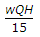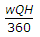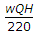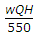# Civil Engineering - Water Supply Engineering - Discussion

### Discussion :: Water Supply Engineering - Section 4 (Q.No.39)

39.

If w is the weight of water per cubic metre, Q is the discharge in cubic metres per sec and H is the total head, the required water horse power of the pump, is

 [A].[B].[C].[D].[E]. none of these.

Explanation:

No answer description available for this question.

 Shahzeb said: (Jun 2, 2016) The correct formula is WQH/75. Plese tell me if I'm wrong.

 Sagar said: (Feb 27, 2017) Agree @Shahzeb. It is Wqh/75.

 Farhan said: (Oct 3, 2017) Yes, it should be 75 in place of 15.

 Mrinal Kanti Biswas said: (Nov 14, 2017) If w = unit wt of water in KN/cum. Q = Discharge in cum/sec. H = total head. Then, required H.P. = wQH/0.735.

 Gubendhiran said: (Jan 3, 2018) Hers, H.P = (wQH)/(0.735*efficiency of pump).

 Joe said: (Jan 25, 2020) Water horse power= w*Q*H/75. Break horse power= w*Q*H/ efficiency.

 Pavan said: (Apr 24, 2020) Yeah, it's WQH/75. Not 15.

 Rosie said: (Sep 30, 2021) No it Should be 75 instead of 15.